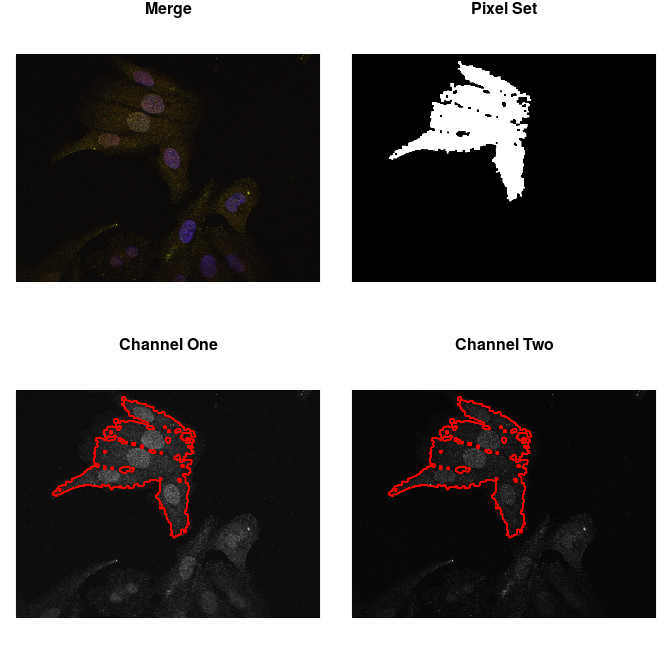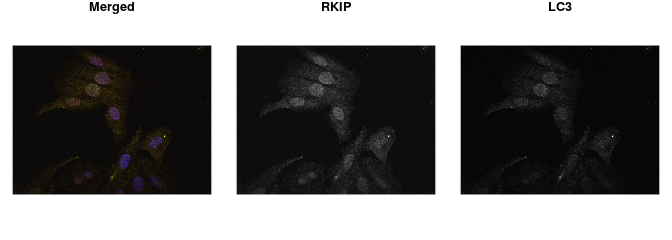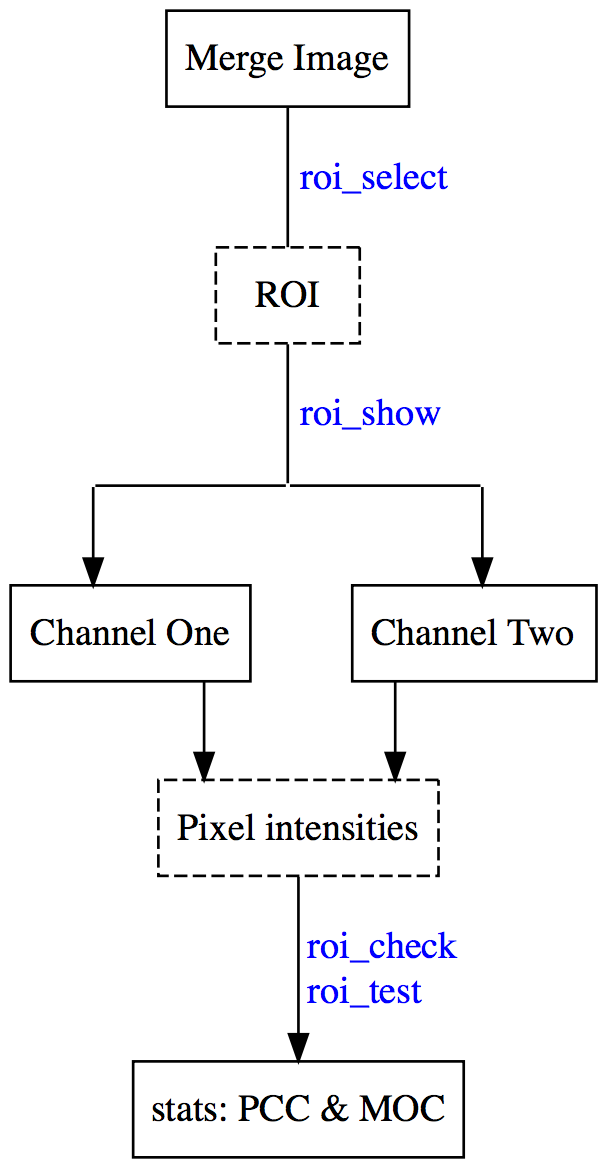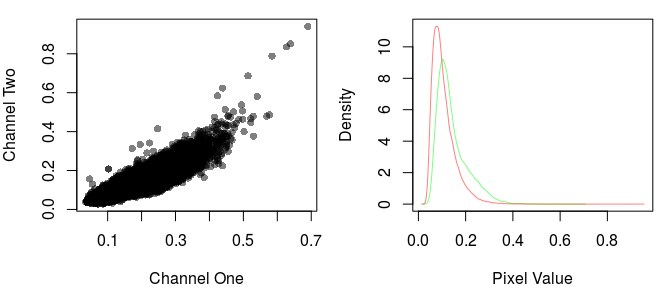# Using colocr

## Overview

A few R packages are available for conducting image analysis, which is a very wide topic. As a result, some of us might feel at a loss when all they want to do is a simple co-localization calculations on a small number of microscopy images. This package provides a simple straight forward workflow for loading images, choosing regions of interest (ROIs) and calculating co-localization statistics. Included in the package, is a shiny app that can be invoked locally to interactively select the regions of interest in a semi-automatic way. The package is based on the R package `imager`.

## Getting started

To get started, load the required packages and the images. The images below are from DU145 cell line and were stained for two proteins; RKIP and LC3. Then, apply the appropriate parameters for choosing the regions of interest using the `roi_select`. Finally, check the appropriateness of the parameters by highlighting the ROIs on the image.

``````# load libraries
library(colocr)

fl <- system.file('extdata', 'Image0001_.jpg', package = 'colocr')

# select ROI and show the results
par(mfrow = c(2,2), mar = rep(1, 4))

img %>%
roi_select(threshold = 90) %>%
roi_show()``````The same can be achieved interactively using an accompanying shiny app. To launch the app run.

``colocr_app()``

The rest of the analysis depends on the particular kind of images. Now, `colocr` implements two simple co-localization statistics; Pearson’s Coefficient Correlation (PCC) and the Manders Overlap Coefficient (MOC).

To apply both measures of correlation, we first get the pixel intensities and call `roi_test` on the merge image.

``````# calculate co-localization statistics
img %>%
roi_select(threshold = 90) %>%
roi_test(type = 'both')
#>         pcc      moc
#> 1 0.9049503 0.983385``````

The same analysis and more can be conducted using a web interface for the package available here

## Detailed Example

The following example uses images from the DU145 prostate cancer cell line. In this experiment, the cell line was treated with probes for two proteins RKIP and LC3. The aim of this experiment is to determine, how much of the two proteins are co-localized or co-distributed in the particular cell line.

``````# load required libraries
library(colocr)

img <- image_load(system.file('extdata', 'Image0001_.jpg', package = 'colocr'))       # merge
img1 <- imager::channel(img, 1)  # red
img2 <- imager::channel(img, 2)  # green

# show images
par(mfrow = c(1,3), mar = rep(1,4))
plot(img, axes = FALSE, main = 'Merged')
plot(img1, axes = FALSE, main = 'RKIP')
plot(img2, axes = FALSE, main = 'LC3')``````The `colocr` package provides a straight forward workflow for determining the amount of co-localization. This workflow consists of two steps only:

• Choosing regions of interest (ROI)
• Calculating the correlation between the pixel intensities

The first step can be achieved by calling `roi_select` on the image. In addition, `roi_show` can be used to visualize the regions that were selected to make sure they match the expectations. Similarly, `roi_check` can be used to visualize the pixel intensities of the selected regions. The second step is calling `roi_test` to calculate the co-localization statistics.

The calls to these functions can be piped using `%>%` to reduce the amount of typing and make the code more readable.### Choosing ROIs

The function `roi_select` relies on different algorithms from the `imager` package. However, using the functions to select the ROIs doesn’t require any background knowledge in the workings of the algorithms and can be done through trying different parameters and choosing the most appropriate ones. Typically, one wants to select the regions of the image occupied by a cell or a group of cells. However, the package can also be used to select certain areas/structures within the cell if they are distinct enough. By default, the largest contiguous region of the image is selected, more regions can be added using the argument `n`. The details of the other inputs are documented in the function help page `?roi_select`.

``````# select regions of interest
img_rois <- img %>%
roi_select(threshold = 90)``````

This function returns `cimg` object containing the original input image and an added attribute called `label` which indicates the selected regions. `label` is a vector of `integer`s; with 0 indicating the non-selected pixels and 1 for the selected regions. When the argument `n` is provided to `roi_select`, 1 is replaced by `integer` labels for each of the selected regions separately.

``````# class of the returned object
class(img); class(img_rois)
#>  "cimg"         "imager_array" "numeric"
#>  "cimg"         "imager_array" "numeric"

# name of added attribut
names(attributes(img)); names(attributes(img_rois))
#>  "class" "dim"
#>  "class" "dim"   "label"

# str of labels
label <- attr(img_rois, 'label')
str(label)
#>  num [1:480000] 0 0 0 0 0 0 0 0 0 0 ...

# unique labels
unique(label)
#>  0 1``````

Now, to make sure these parameters are appropriately encompassing the ROIs, call the `roi_show` to visualize side by side the original merge picture, a low resolution picture of the ROI and the images from the two different channels highlighted by the ROIs.

``````# select ROI and show the results
par(mfrow = c(2,2), mar = rep(1, 4))

img_rois %>%
roi_show()``````Both the co-localization statistics implemented in this package quantify different aspects of the linear trend between the pixel intensities from the two channels of the image. Therefore, it is useful to visualize this trend and the distribution of the intensities to make sure the analysis is appropriate.

``````# show the scatter and density of the pixel values
par(mfrow=c(1,2), mar = c(4,4,1,1))

img_rois %>%
roi_check()``````Arguably, selecting the regions of interest is the most time consuming step in this kind of analysis. Usually, one has to do this selection by hand when using image analysis software such as imageJ. This package only semi-automates this step, but still relies on the user’s judgment on which parameters to use and whether or not the selected ROIs are appropriate. To make life easier, the package provides a simple shiny app to interactively determine these parameters and use it in the rest of the workflow. To launch the app run the following

``````# run the shiny app
colocr_app()``````

And here is a screen shot from the app after applying the same parameters used previously.